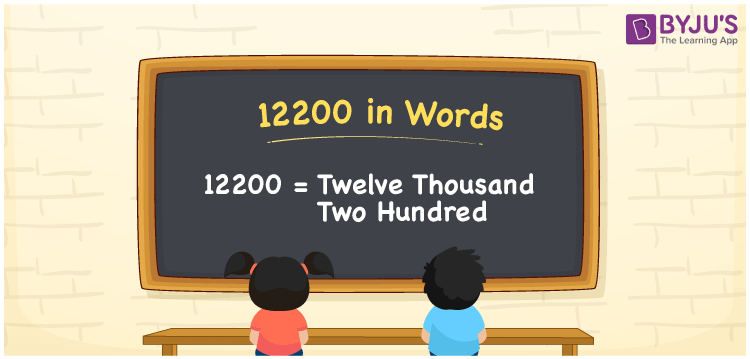# 12200 in Words

12200 in words is written as “Twelve thousand two hundred”. For example, a cheque of Rs.12200 is written as Rupees Twelve thousand two hundred only. In Maths, 12200 is a cardinal number that expresses a quantity. Learn more about Numbers In Words and writing the number names in English at BYJU’S.

 12200 in Words Twelve thousand two hundred Twelve thousand two hundred in Numerical Form 12200

## 12200 in English Words## How to Write 12200 in Words?

The number 12200 in words can be written using a place value chart. Since 12200 is a five-digit number, thus,

 Ten-thousand Thousands Hundreds Tens Ones 1 2 2 0 0

From the above table,

1 → Ten thousands

2 → Thousands

2 → Hundreds

0 → Tens

0 → Ones

Hence, when we read the number from the right to left, it is Twelve thousand two hundred.

### Expanded Form of 12200

We can write the expanded form as:

1 x Ten thousand + 2 x Thousand + 2 × Hundred + 0 × Ten + 0 × One

= 1 x 10000 + 2 x 1000 + 2 × 100 + 0 × 10 + 0 × 1

= 10000 + 2000 + 200 + 0 + 0

= 10000 + 2000 + 200

= Twelve thousand two hundred

12200 is a whole number that is succeeded by 12199 and preceded by 12201. Learn more about the number 12200 below:

• 12200 in Words – Twelve thousand two hundred
• Is 12200 an odd number? – No
• Is 12200 an even number? – Yes
• Is 12200 a perfect square number? – No
• Is 12200 a perfect cube number? – No
• Is 12200 a prime number? – No
• Is 12200 a composite number? – Yes

## Frequently Asked Questions on 12200 in words

Q1

### What is 12200 in words?

12200 in words is given by Twelve thousand two hundred.
Q2

### What is the rule to write 12200 in words?

To write the 12200 in words, we should use the place value rule, where the position of each digit helps in determining the name of the number.
Q3

### What is the value of 12200 + 100 in words?

12200 + 100 = 12300, i.e., Twelve thousand three hundred in words.
Test your Knowledge on 12200 in Words